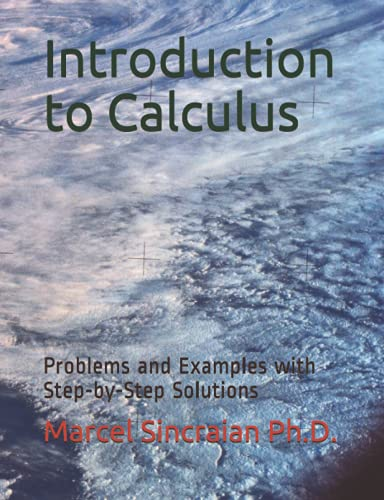# Introduction to Calculus: Problems and Examples with Step-by-Step Solutions

• Length: 349 pages
• Edition: 1
• Publisher:
• Publication Date: 2021-05-01
• ISBN-10: 1777502241
• ISBN-13: 9781777502249
Description

This book provides students with a tool to improve their knowledge in preparation to learning Calculus. This is done in a light hearted manner in order to help students having fun while preparing to understand Calculus. This book is intended to be a quick review of the introduction to Calculus. The book follows the main precalculus concepts needed in order to understand Calculus. It starts with the Cartesian System, and then it continues with Geometry and Trigonometric ratios, Algebra. From introduction to powers, and logarithms to polynomials, graphs of linear equations and quadratic equations. Then it follows Calculus I curriculum for high schools. Chapter 5 is a review of Functions. Chapter 6 is about Limits; Chapter 7 deals with Differentiation, and Chapter 8 is all about Integrals.